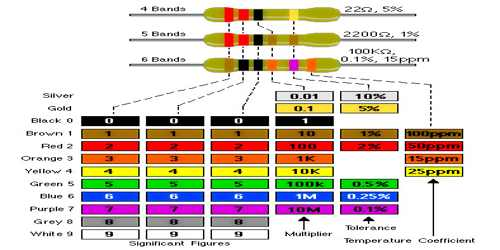The Color Codes used on resistors in carbon, carbon film and metal film types are widely used and a ‘must learn’ for electronics engineers. Resistors are usually very tiny and it is difficult to print resistance values on them. So, color bands are printed on them to represent the electrical resistance. These color bands are known as resistor color code.

Resistors are available in a range of different resistance values from fractions of an Ohm (Ω) to millions of Ohms.

Values of resistance, tolerance, and wattage rating are generally printed onto the body of the resistor as numbers or letters when the resistor’s body is big enough to read the print, such as large power resistors. In most cases, when the resistor is small such as a 1/4 W carbon or film type, these specifications will be color-coded. These colored painted bands produce a system of identification generally known as Resistor Color Code.

All leaded resistors with a power rating up to one watt are marked with color bands. They are given by several bands and together they specify the resistance value, the tolerance rate, and sometimes the reliability or failure rates. The number of bands present in a resistor varies from three to six.

The tolerance values represent by how much the resistance can vary from its mean value in terms of percentage. A gold band represents the lowest variation, so be sure to buy these at the electronics store.  The value of the given resistance is: 22 Ω ± 5% . The tolerance of the resistor can be calculated as follows:

Tolerance=Value of resistor × value of tolerance band

= 22 Ω × 5% = 1.1 Ω

This means that the 22 Ω resistors with a tolerance value of 1.1 Ω could range from the actual value as much as 23.1 Ω to as little as 20.9 Ω. It is important to note that the band next to the tolerance band represents the multiplier. All the bands to the left of this band represent the significant digits. There can be more than two such bands.

The humble resistor is a key component of many renewable energy systems. They are designed to resist electricity and can, therefore, be used to supply the correct voltage or a suitably limited current to the various components in an electric circuit. Because resistors are so small too small to print numerical resistance values on they are instead marked with four, five, or (less commonly) six colored bands. Each color represents a different number and so the value (in Ohms) of the resistor can easily be calculated.

An international resistor color code scheme was developed many years ago as a simple and quick way of identifying a resistor’s ohmic value no matter what its size or condition. It consists of a set of individual colored rings or bands in spectral order representing each digit of the value of the resistor.

The resistor color code markings are always read one band at a time starting from the left to the right, with the larger width tolerance band oriented to the right side indicating its tolerance. By matching the color of the first band with its associated number in the digit column of the color chart below the first digit is identified and this represents the first digit of the resistance value.

Resistor Color Code Chart –Colors on the resistor like brown, red, green, blue, and violet are used as tolerance codes on 5-band resistors only. The blank (20%) “band” is only used with the “4-band” code (3 colored bands + a blank “band”).

Following are the ways to understand which end of the resistor must be used for reading the color codes:

Most of the resistors have color bands grouped closer at one end. Hold the resistor with these grouped bands to our left. Always read the resistor from left to right.

Resistors never have a metallic band on the left. If the resistor has either gold or silver band on either end, then the tolerance of the resistor is 5% or 10%. This band of the resistor must be position on the right and the color code must be read from left to right.

Resistors range from 0.1 Ohm to 10 Mega-ohms. For a four-band resistor, the third color will always be blue i.e. 106 or less and for a five-band resistor, the fourth color will always be green i.e. 105 or less.

There are many mnemonic phrases to help us to remember the order of the colors:

Black Brown Red Orange Yellow Green Blue Violet Gray White…which correspond to the values 0-9.

Information Sources: